Learn about tree, tree traversal, binary heap, trie and their running time.

# Tree

• Tree is a data structure composed of nodes.
• Each tree has a root node.
• The root node has zero or more child nodes.
• Each child node has zero or more child nodes, and so on.
• Features of tree
• Tree cannot contain cycles.
• The nodes may or may not be in a particular order.
• They may or may not have links back to their parent nodes.

## Trees vs. Binary Trees

• Binary tree is a tree in which each node has up to two children.
• Other than that, it will be $n$-ary tree.
• A node is called a leaf node if it has no children.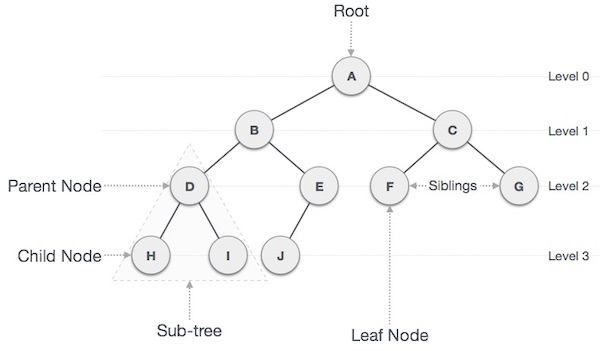## Binary Tree vs. Binary Search Tree

• Binary search tree is a binary tree in which every node fits a specific ordering property:
• For each node $n$, $% $
• In some definitions, the tree cannot have duplicate values.
• In others, the duplicate values will be on the right or can be on either side.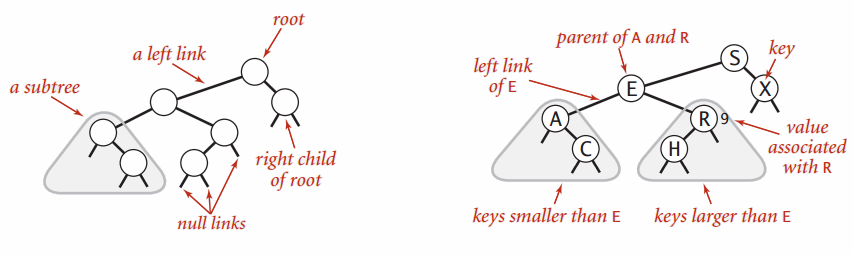## Balanced vs. Unbalanced

• In order to guarantee that $H = \log N$ ($H$:height, $N$: number of nodes), we can force the tree to be height-balanced.
• Relation between $H$ and $N$ in a tree can vary from $H=N$ (degenerate tree) to $H=\log N$.
• Two common types of balanced trees are red-black trees and AVL trees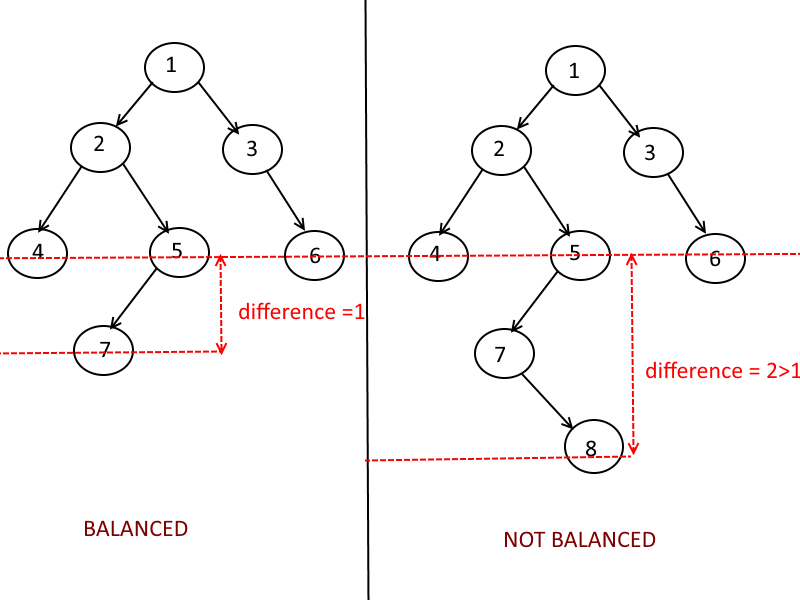## Complete Binary Trees

• Complete binary tree is a binary tree in which every level of the tree is fully filled, except for perhaps the last level.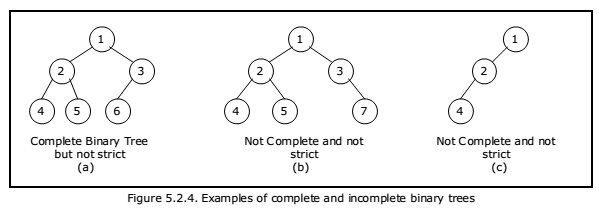## Full Binary Trees

• Full binary tree is a binary tree in which every node has either zero or two children, no nodes have only one child.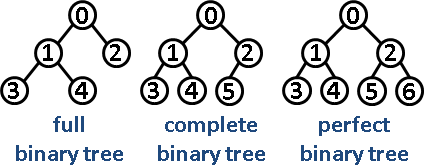## Perfect Binary Trees

• Perfect binary tree is one that is both full and complete.
• A perfect tree must have exactly $2^k-1$ nodes where $k$ is the number of levels.

# Binary Tree Traversal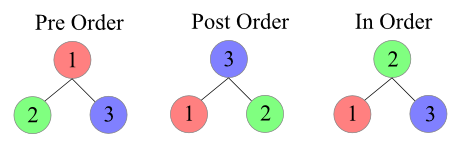## Pre-Order Traversal

• In a pre-order traversal, the root of tree is visited first and then the subtrees rooted at its children are traversed recursively.
• Time complexity: $O(n)$, where $n$ is the number of positions in the tree.
Algorithm preorder(T, p):
perform the "visit" action for position p
for each child c in T.children(p) do
preorder(T,c) # recursively traverse the subtree rooted at c


## Post-Order Traversal

• In a post-order traversal, it recursively traverses the subtrees rooted at the children of the root first, and then visits the root.
• Time complexity: $O(n)$, where $n$ is the number of positions in the tree.
Algorithm postorder(T, p):
for each child c in T.children(p) do
postorder(T,c) # recursively traverse the subtree rooted at c
perform the "visit" action for position p


## In-Order Traversal

• In a in-order traversal, for every position p, the inorder traversal visits p after all the positions in the left subtree of p and before all the positions in the right subtree of p.
• Time complexity: $O(n)$, where $n$ is the number of positions in the tree.
Algorithm inorder(p):
if p has a left child lc then
inorder(lc) # recursively traverse the left subtree of p
perform the "visit" action for position p
if p has a right child rc then
inorder(rc) # recursively traverse the right subtree of p


# Binary Heaps (Min-Heaps and Max-Heaps)

• Min-heap is a complete binary tree where each node is smaller than its children.
• The root is the minimum element in the tree.
• Max-heap is essentially equivalent but the elements are in descending order rather than ascending order.

## Insert

• Insert element at the rightmost spot, then we fix the tree by swapping the new element with its parent, until we find an appropriate spot for the element.
• Takes $O(\log n)$ time, where $n$ is the number of nodes in the heap.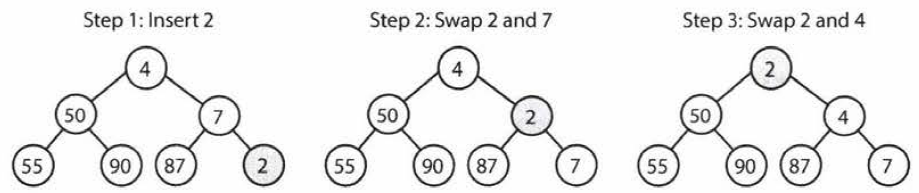## Extract Minimum Element

• First, we remove the minimum element and swap it with the last element in the heap.
• Then, we bubble down this element, swapping it with one of its children until the min-heap property is restored.
• Takes $O(\log n)$ time, where $n$ is the number of nodes in the heap.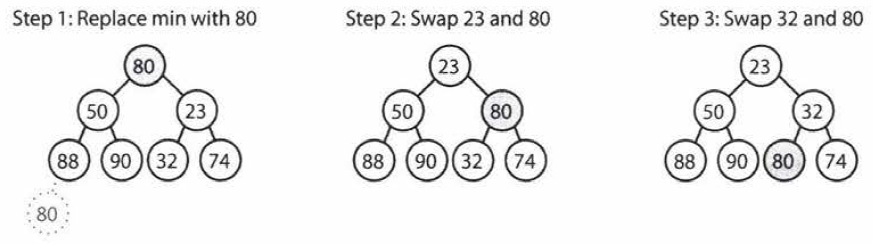## Analysis of a Heap-Based Priority Queue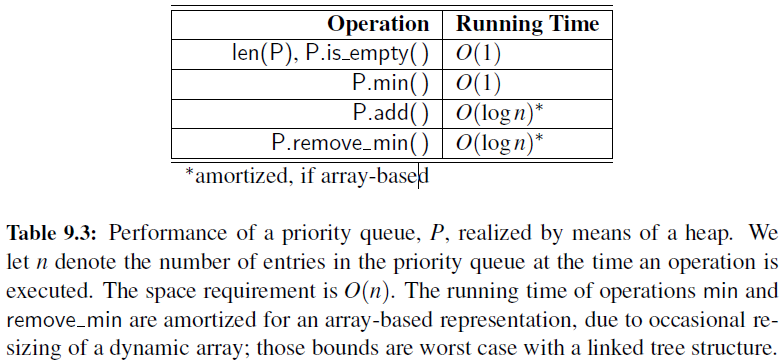## Python’s heapq Module

• Python’s standard distribution includes a heapq module that provides support for min-heap priority queues.
• Functions: heappush(L,e), heappop(L), heappushpop(L,e), heapreplace(L,e), heapify(L), nlargest(k,iterable), nsmallest(k,iterable)

# Tries (Prefix Trees)

• Trie is a variant of an n-ary tree in which characters are stored at each node.
• Each path down the tree may represent a word.
• The * nodes (null nodes) are often used to indicate complete words.
• A node in a trie could have anywhere from 1 through $\text{alphabet_size}+1$ children (or, 0 if a boolean flag is used instead of a * node).
• A trie can check if a string is a valid prefix in $O(k)$ time, where $k$ is the length of the string.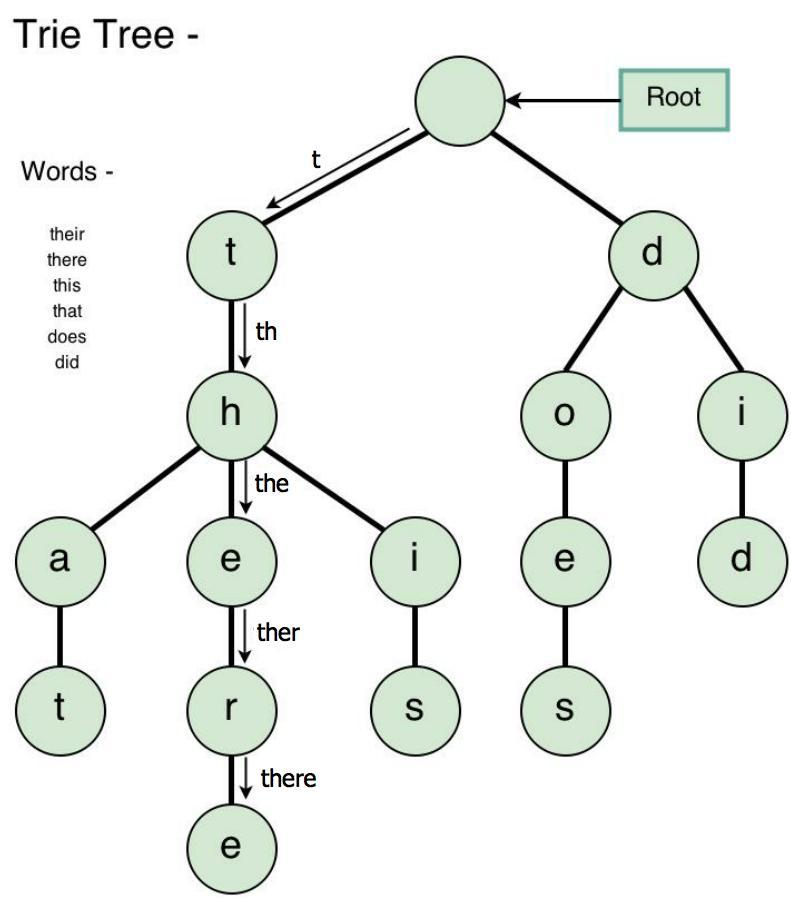• Book: Cracking the coding interview [Link]
• Book: Data structures and algorithms in python [Link]